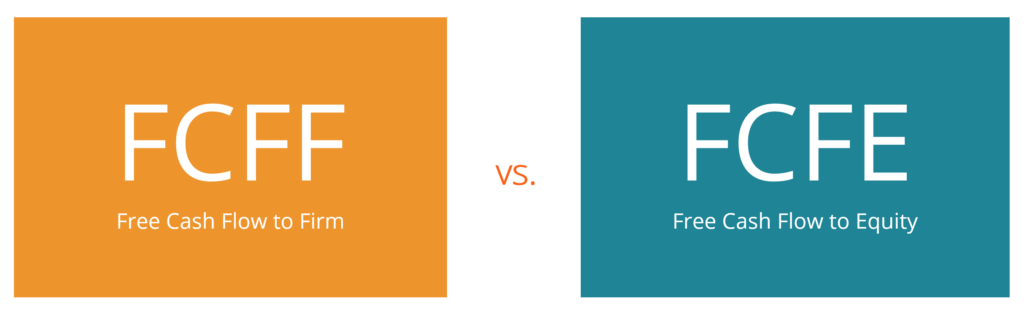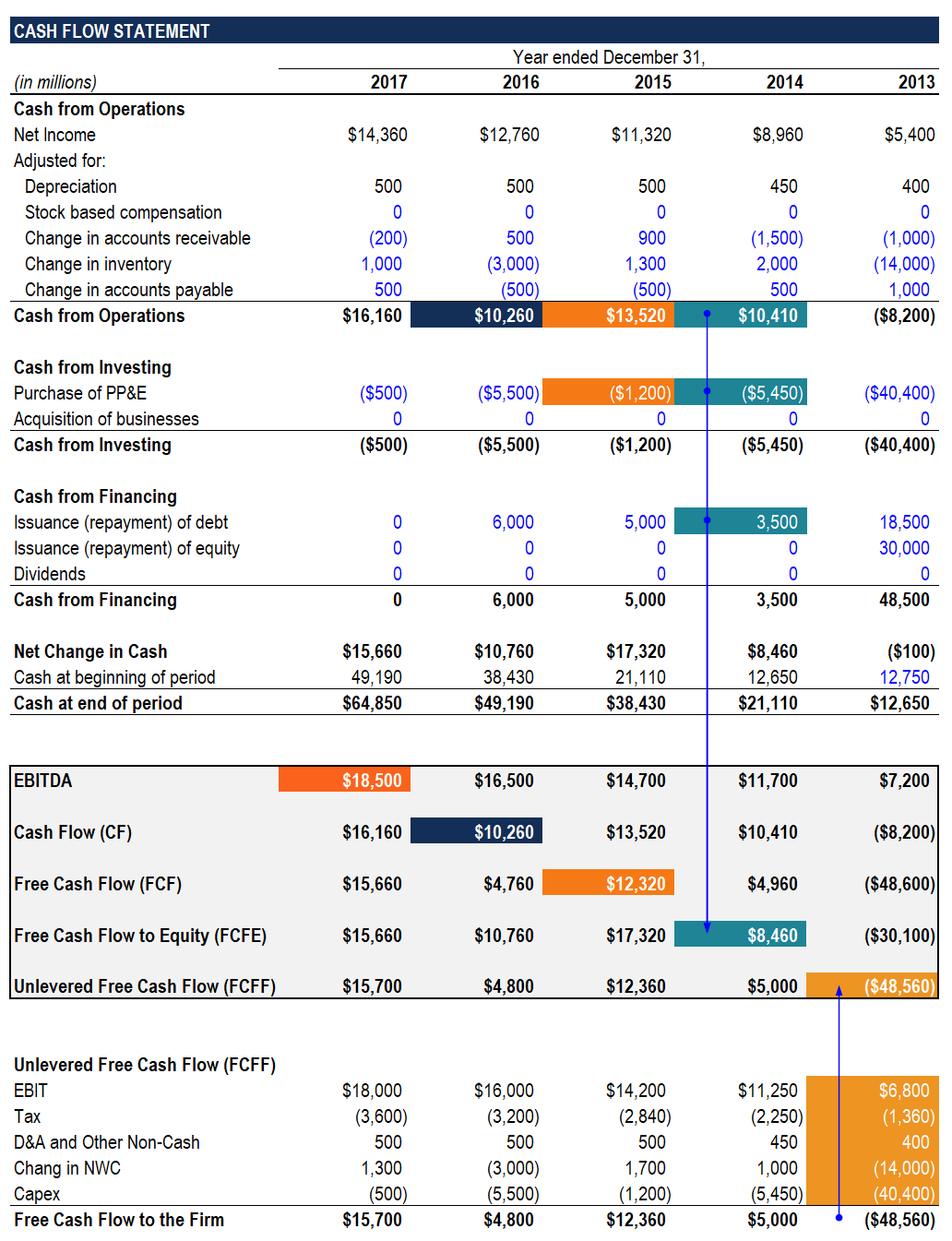# FCFF vs FCFE

Differences between Free Cash Flow to Firm (FCFF) and Free Cash Flow to Equity (FCFE)

## FCFF vs FCFE

There are two types of Free Cash Flows: Free Cash Flow to Firm (FCFF) (also referred to as Unlevered Free Cash Flow) and Free Cash Flow to Equity (FCFE), commonly referred to as Levered Free Cash Flow. It is important to understand the difference between FCFF vs FCFE as the discount rate and numerator of valuation multiples depend on the type of cash flow used.### Free Cash Flow

Before looking into the difference between FCFF vs FCFE, it is important to understand what exactly is Free Cash Flow (FCF). Free Cash Flow is the amount of cash flow a firm generates (net of taxes) after taking into account non-cash expenses, changes in operating assets and liabilities, and capital expenditures.

Free Cash Flow is a more accurate metric than EBITDA, EBIT, and Net Income as they leave out large capital expenditures and change in cash due to changes in operating assets and liabilities. Also, metrics such as EBIT and Net Income include non-cash expenses, further misrepresenting the true cash flow of a business.

Due to the reasons mentioned above, Free Cash Flow is often used in a DCF analysis and therefore, a clearer understanding of the concept is important for finance interviews, especially for Investment Banking and Corporate Finance roles.

### Difference between FCFF vs FCFE

The key difference between Unlevered Free Cash Flow and Levered Free Cash Flow is that Unlevered Free Cash Flow excludes the impact of interest expense and net debt issuance (repayments) whereas Levered Free Cash Flow includes the impact of interest expense and net debt issuance (repayments).

There are a number of ways to calculate the two cash flows, and questions on the differences and methods of calculation often come up in finance interviews. Given below are a few methods to calculate both Unlevered and Levered Free Cash Flow.### How to Calculate Unlevered Free Cash Flow

• EBIT * (1-Tax Rate) + Non-Cash Expenses – Changes in Operating Assets & Liabilities – CapEx
• Cash Flow from Operations + Tax Adjusted Interest Expense – CapEx
• Net Income + Tax Adjusted Interest Expense + Non-Cash Expenses – Changes in Operating Assets & Liabilities – CapEx

### How to Calculate Levered Free Cash Flow

• Net Income + Non-Cash Charges – Changes in Operating Assets & Liabilities – CapEx + Net Debt Issued (Repaid)
• Cash Flow from Operations – CapEx + Net Debt Issued (Repaid)

### Valuation Multiples: FCFF vs FCFE

While calculating valuation multiples, we often use either Enterprise Value or Equity Value in the numerator with some cash flow metric in the denominator. While we almost always use both Enterprise Value and Equity Value multiples, it is extremely important to understand when to use which. Their usage depends on the metric that is in the denominator. If the denominator includes interest expense, Equity Value is used, and if it does not include interest expense, Enterprise Value is used.

Therefore, Equity Value is used with Levered Free Cash Flow and Enterprise Value is used with Unlevered Free Cash Flow. Enterprise Value is used with Unlevered Free Cash Flows because this type of cash flow belongs to both debt and equity investors. However, Equity Value is used with Levered Free Cash Flow as Levered Free Cash Flow includes the impact of interest expense and mandatory debt repayments, and therefore belongs to only equity investors. Similarly, if EBIT is in the denominator, Enterprise Value would be used in the numerator and if Net Income is in the denominator, Equity Value would be used in the numerator.

### Discount Rate: FCFF vs FCFE

Just like valuation multiples differ depending on the type of cash flow being used, the discount rate in a DCF also differs depending on whether Unlevered Free Cash Flows or Levered Free Cash Flows are being discounted.

If Unlevered Free Cash Flows are being used, the firm’s Weighted Average Cost of Capital (WACC) is used as the discount rate because one must take into account the entire capital structure of the company. Calculating Enterprise Value means including the share of all investors.

If Levered Free Cash Flows are used, the firm’s Cost of Equity should be used as the discount rate because it involves only the amount left for equity investors. It ensures calculating Equity Value instead of Enterprise Value.

### Conclusion

Understanding the differences between Unlevered Free Cash Flows and Levered Free Cash Flows, along with when to use which method, is extremely important for most entry-level Corporate Finance and Investment Banking roles. While questions on this topic can sometimes get tricky, gaining an understanding of the concepts mentioned above is a good starting point.

Thank you for reading this guide on FCFF vs FCFE. CFI offers the Financial Modeling & Valuation Analyst (FMVA) certification program for financial analysts. To continue learning and advancing your career, the additional resources below will be helpful:

• Enterprise Value vs Equity Value
• How the 3 Financial Statements are Linked
• The Ultimate Cash Flow Guide
• Weighted Average Cost of Capital (WACC)

### Valuation Techniques

Learn the most important valuation techniques in CFI’s Business Valuation course!

Step by step instruction on how the professionals on Wall Street value a company.

Learn valuation the easy way with templates and step by step instruction!